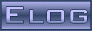Back Midas Rome Roody Rootana
 Midas DAQ System Not logged inMessage ID: 2229     Entry time: 24 Jun 2021     Reply to this: 2231   2234   2253
 Author: Konstantin Olchanski Topic: Bug Fix Subject: changes in history plots
```I am updating the history plots. Main changes:

- the old history display code should again be easily usable (use the "open in old history display" checkbox)
- the history plot editor has an "edit in ODB" button that takes as to the plot definition in ODB (sometimes it is
easier to editing things in the ODB editor)
- error in history plot editor that created "formula" entry of incorrect size should be fixed
- "reorder" (and "delete entry") functions in the history plot editor should work again (plus added explanation text)
- "factor" and "offset" restored in the history plot editor
- added the long desired "voffset" to simplify plot scaling and positioning
- (factor, offset and voffset do not yet work in the new history plots, TBI ASAP)
- history plot editor and generate_hist_graph() now use the same code to read plot definitions from ODB. There should
be no more confusion about content of history plot entries in ODB and what each entry is supposed to do.

These changes have been precipitated by our inability to plot high voltage voltage and current on the same plot,
see bug https://bitbucket.org/tmidas/midas/issues/308/history-plot-formula-cannot-be-used-to

Voltage is in the range 0..1000 (volts) and current is in the range 0..50 and 0..0.100, autoscaling on voltage
makes the currents invisible at the zero line. In the past, we used the "factor" setting to scale
the graphs so we can see both voltage and currents at the same time (currents scaled up by factor 25 and 600,
as example).

The new "formula" feature was supposed to replace (and improve upon) the "factor" and "offset". But if I use
the formula "x*25", suddenly the plot is telling us that current values are not 50 uA, but 1250 uA (50*25),
and this is just wrong. We do not want to scale the micro-amps, we want to better position the plot on the graph,
like the old "factor" and "offset" allowed us to do.

So the idea is to use this computation:

y_position_on_plot = offset + factor*(formula(history_value) - voffset)

- "formula" is to transform history values into physical values (i.e. pressure meter reports bars, but we want atm, or
voltmeter is reading in discrete units of 0.125V, we want to see volts)
- "factor" and "offset" is to position the graphs on the plot for best visual presentation of data
- I also added is the much desired "voffset", you only know it is needed if you have a non-zero "offset" and you need
to change the "factor", surprise, "offset" has ot be changed, too, and good luck recalculating it correctly in one
try.

The way to use this stuff:
- adjust "voffset" to bring the graph to around y=0
- increase the "factor" to zoom-in on features and stuff
- adjust "offset" to move the graph up and down relative to all the other graphs on the plot
- now one can zoom in and out as needed by changing the "factor" and the plot will stay roughly in the right place
without having to readjust the offsets.

K.O.```
ELOG V3.1.4-2e1708b5